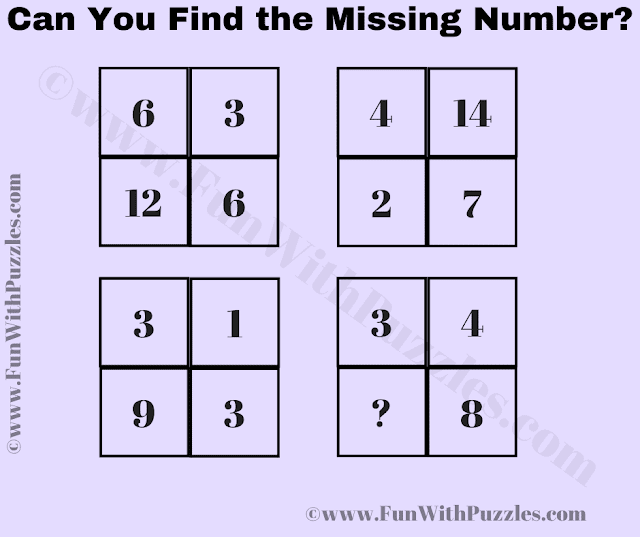Sharpen your logical reasoning and mathematical skills with this easy logic puzzle. Decode the relationships between numbers in four squares to find the missing number and test your puzzle-solving abilities.

Embark on a journey of logical exploration with our engaging logic puzzle, "Crack the Code Squares." Designed to challenge your logical reasoning and mathematical prowess, this puzzle presents a grid of four squares, each containing four numbers. Your task is to uncover the intricate relationships hidden within the numbers and solve the mystery of the missing number in the final square.Logical Math Puzzle: Find the Missing Number

As you examine each square, your mission is to unveil the logical formula that connects the numbers. By applying your analytical skills and logical reasoning, you can deduce the pattern that underlies the sequence of numbers. With the formula in hand, your next step is to identify the missing number that adheres to the established pattern. This intriguing puzzle is a fantastic exercise for both your logical thinking and mathematical abilities.

Tailored for school-going students, this logic brain teaser offers an enjoyable challenge that encourages critical thinking. While the puzzle is designed to be accessible, it still demands a keen eye for patterns and a logical approach. If you successfully crack the puzzle, don't hesitate to share your answer and the logical reasoning behind it in the comments section. Engage in an exchange of ideas with fellow puzzle enthusiasts and showcase your puzzle-solving skills.

Get ready to unravel the mysteries of the code-cracking squares and witness the satisfaction of deciphering logical patterns and numbers. This puzzle promises an intellectual adventure that rewards your efforts with a deeper understanding of logic and mathematics.

The answer to this "Missing Number Puzzle", can be viewed by clicking on the answer button.

Unknown said...

andwer is 6
6x6=36
36/3=12

4x7=28
28/14=2

3x3=9
9/1=9

3x8=24
24/4=6